# Kähler manifold

A complex manifold which admits a Kähler metric. Sometimes it is called a manifold of Kähler type, with the term "Kähler manifold" being reserved for manifolds actually equipped with a Kähler metric . Any submanifold of a Kähler manifold is a Kähler manifold. In particular, all projective complex algebraic varieties without singular points are Kähler manifolds, and, moreover, their Kähler metric is induced by the Fubini–Study metric on the complex projective space. Similarly, every submanifold in the affine space(in particular, every Stein manifold) is a Kähler manifold. Other examples of Kähler manifolds are obtained if one considers the quotient spaceof a Kähler manifoldby a discrete groupof analytic automorphisms preserving the Kähler metric. In particular, every complex torus is a Kähler manifold. Any one-dimensional complex manifold is Kählerian.

The theory of harmonic forms on a compact Kähler manifoldyields the following properties of the de Rham and Dolbeault cohomology groups on(see , , and also , where these properties were first established for projective algebraic varieties):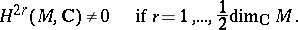Holomorphic forms on a compact Kähler manifold are closed. In particular,whereis the space of all holomorphic-forms on. If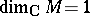, the number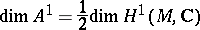is the genus ofas a compact Riemann surface (cf. also Genus of a surface; Riemann surface). The above properties provide the basis for the construction of examples of non-Kähler compact manifolds, the simplest of which is the Hopf surface, which is diffeomorphic to.

A Kähler manifoldis called a Hodge variety if its Kähler metric is a Hodge metric. Any projective algebraic variety without singular points is a Hodge variety relative to the metric induced by the Fubini–Study metric. Conversely, any compact complex manifoldequipped with a Kählerian Hodge metriccan be biholomorphically imbedded in a complex projective space in such a way that the metric induced onby the Fubini–Study metric may be expressed asfor some natural number(Kodaira's projective imbedding theorem , ). Thus, a compact complex manifoldis isomorphic to a projective algebraic variety if and only if it is a Hodge variety. Another form of this criterion: A compact complex manifoldis a projective algebraic variety if and only if it admits a negative vector bundle. Kodaira's theorem can be generalized to complex spaces (see , ). Compact Kähler manifolds which are not Hodge varieties may be found among the two-dimensional complex tori. For example, this is the case for the torus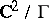, whereis the lattice spanned by the vectors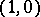,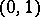,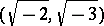,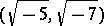(see , ). Another necessary and sufficient condition for an-dimensional compact Kähler manifoldto be projective is that there existalgebraically independent meromorphic functions on.

Any non-compact complete Kähler manifold with positive sectional curvature is a Stein manifold. The same is true of any simply-connected complete Kähler manifold of non-positive sectional curvature .

How to Cite This Entry:
Kähler manifold. Encyclopedia of Mathematics. URL: http://encyclopediaofmath.org/index.php?title=K%C3%A4hler_manifold&oldid=18475
This article was adapted from an original article by A.L. Onishchik (originator), which appeared in Encyclopedia of Mathematics - ISBN 1402006098. See original article